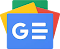## Saturday April 22 2023Scientific computer with functions, which can be used especially by high school students or college students.

It offers you a wide range of tools to make your calculations easier and faster.

Its features include the ability to evaluate complex expressions with parentheses, variables, constants, functions, calculation in four systems, binary, octal, decimal, hexadecimal, transcendental functions, graphs of functions, calculations with polynomials and fractions, and more.

CHARACTERISTICS

• Complex mathematical expressions with parentheses, variables, constants and functions.
• Real numbers.
• Complex numbers.
• Fractions (real or complex).
• Calculations available in four number systems: binary, octal, decimal, hexadecimal.
• Number system conversions (including decimal numbers).
• Wide range of arithmetic, trigonometric and hyperbolic functions.
• Graphing functions with multiple functions on the same graph.
• Derivative of a function (differentiation).
• Higher order derivatives.
• Some derivatives.
• Polynomials.
• Addition, subtraction and multiplication of polynomials.
• Division of polynomials (polynomial division with remainder).
• Raising polynomials to power.
• Convert units (length, speed, mass, volume, temperature, etc.)
• Variations with / without repetition.
• Combinations with / without repetition.Follow us on Google News